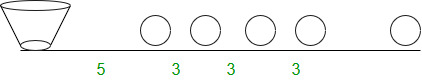# Find the distance covered to collect items at equal distances

A race is going on in which several stones are placed on a road. A bucket is placed at the starting point of the race, which is 5 units away from the first stone. The other stones are 3 units apart from each other and lie straight in a line one after another. I.e., the distance between 1st and 2nd stone is 3 units, between 3rd and 4th stone, it is also 3 units and so on. The competitor starts from the bucket, picks up the nearest stone, goes back and puts that stone into the bucket, then runs again to collect the next nearest stone, runs back, and puts it in the bucket. This way the process is continued till all the stones have been put into the bucket.

Now if there are n stones lying on the ground, how much distance will be covered by the competitor in completing the whole process.Examples:

```Input : n = 3
Output : Distance = 48
Explanation
= 2*5 + 2(5 + 3) + 2(5 + 3 + 3)
= 10 + 16 + 22
= 48

Input : n = 5
Output : Distance = 110
Explanation
= 2*5 + 2(5 + 3) + 2(5 + 3 + 3) + 2(5 + 3 + 3 + 3) + 2(5 + 3 + 3 + 3 + 3)
= 10 + 16 + 22 + 28 + 34
= 110
```

## Recommended: Please try your approach on {IDE} first, before moving on to the solution.

Observing the pattern:

Distance run by competitor to pick first stone = 2 * 5
Distance run by competitor to pick second stone = 2(5 + 3)

Distance run by competitor to pick third stone = 2(5 + 3 + 3)
= 2(5 + (2 * 3))

Distance run by competitor to pick fourth stone = 2(5 + 3 + 3 + 3)
= 2(5 + (3 * 3))

Distance run by competitor to pick fifth stone = 2(5 + 3 + 3 + 3 + 3)
= 2(5 + (4 * 3))
.
.
.

Distance run by competitor to pick n-th stone = 2(5 + 3 + 3 + ……. + (n-1) times )
= 2(5 + (n-1) *3)

So total distance run by competitor = sum of all the above distances
= (2 * 5) + 2(5 + 3) + 2(5 + (2 * 3)) + 2(5 + (3 * 3)) + ………….. + 2(5 + (n-1) *3)
= 2(5 + (5 + 3) + (5 + (2 * 3)) + (5 + (3 * 3)) + ………………. + (5 + (n-1) * 3)
= 2(5 + 5 + 5 …… + n times) + (3 + (2 * 3) + (3 * 3) + ……… + (n-1) * 3)
= 2(5n + 3(1 + 2 + 3 + ……………. + n-1))
= 2(5n + 3/2[(n-1)*(n-1 + 1)] )
= 2(5n + 3/2[(n-1)*n])
= 2(5n + 3/2(n2 – n))
= 10n + 3*n2 – 3*n
= 3*n2 + 7*n
= n*((3 * n) + 7)

Below is the implementation of the approach:

## C++

 `// C++ program to calculate ` `// the distance for given problem ` `#include ` `using` `namespace` `std; ` ` `  `// function to calculate the ` `// distance ` `int` `find_distance(``int` `n) ` `{ ` `    ``return` `n * ((3 * n) + 7); ` `} ` ` `  `// Driver program ` `int` `main() ` `{ ` `    ``int` `n = 5; ` `    ``cout << ``"Distance = "` `<< find_distance(n); ` `    ``return` `0; ` `} `

## Java

 `// Java program to calculate the ` `// distance for given problem ` `class` `demo { ` ` `  `    ``// function to calculate ` `    ``// the distance ` `    ``public` `static` `int` `find_distance(``int` `n) ` `    ``{ ` `        ``return` `n * (``3` `* n + ``7``); ` `    ``} ` ` `  `    ``// Driver program ` `    ``public` `static` `void` `main(String args[]) ` `    ``{ ` `        ``int` `n = ``5``; ` ` `  `        ``System.out.print(``"Distance = "``); ` `        ``System.out.println(find_distance(n)); ` `    ``} ` `} `

## Python3

 `# Python3 code to calculate ` `# the distance for given problem ` ` `  `# function to calculate the ` `# distance ` `def` `find_distance(n): ` `     `  `    ``return` `n ``*` `((``3` `*` `n) ``+` `7``) ` ` `  `# main function ` `n ``=` `5` `ans ``=` `find_distance( n ) ` `print` `(ans) ` ` `  `# This code is contributed by Saloni Gupta `

## C#

 `// C# program to calculate ` `// the distance for given problem ` `using` `System; ` ` `  `class` `GFG { ` ` `  `    ``// function to calculate the ` `    ``// distance ` `    ``public` `static` `int` `find_distance(``int` `n) ` `    ``{ ` `        ``return` `n * ((3 * n) + 7); ` `    ``} ` ` `  `    ``// Driver program ` `    ``public` `static` `void` `Main() ` `    ``{ ` `        ``int` `n = 5; ` ` `  `        ``Console.Write(find_distance(n)); ` `    ``} ` `} ` ` `  `// This code is contributed by ` `// Smitha Dinesh Semwal `

## PHP

 ` `

Output:

```110
```

Attention reader! Don’t stop learning now. Get hold of all the important DSA concepts with the DSA Self Paced Course at a student-friendly price and become industry ready.

My Personal Notes arrow_drop_upCheck out this Author's contributed articles.

If you like GeeksforGeeks and would like to contribute, you can also write an article using contribute.geeksforgeeks.org or mail your article to contribute@geeksforgeeks.org. See your article appearing on the GeeksforGeeks main page and help other Geeks.

Please Improve this article if you find anything incorrect by clicking on the "Improve Article" button below.

Article Tags :
Practice Tags :

Be the First to upvote.

Please write to us at contribute@geeksforgeeks.org to report any issue with the above content.